# 11.1 Solutions by graphing

 Page 1 / 1
This module is from Elementary Algebra by Denny Burzynski and Wade Ellis, Jr. Beginning with the graphical solution of systems, this chapter includes an interpretation of independent, inconsistent, and dependent systems and examples to illustrate the applications for these systems. The substitution method and the addition method of solving a system by elimination are explained, noting when to use each method. The five-step method is again used to illustrate the solutions of value and rate problems (coin and mixture problems), using drawings that correspond to the actual situation.Objectives of this module: be able to recognize a system of equations and a solution to it, be able to graphically interpret independent, inconsistent, and dependent systems, be able to solve a system of linear equations graphically.

## Overview

• Systems of Equations
• Solution to A System of Equations
• Graphs of Systems of Equations
• Independent, Inconsistent, and Dependent Systems
• The Method of Solving A System Graphically

## Systems of equations

A collection of two linear equations in two variables is called a system of linear equations in two variables , or more briefly, a system of equations . The pair of equations

$\left\{\begin{array}{r}5x-2y=5\\ \text{\hspace{0.17em}}x\text{\hspace{0.17em}}\text{\hspace{0.17em}}+\text{\hspace{0.17em}}\text{\hspace{0.17em}}y=8\end{array}$
is a system of equations. The brace $\left\{$ is used to denote that the two equations occur together (simultaneously).

## Solution to a system

We know that one of the infinitely many solutions to one linear equation in two variables is an ordered pair. An ordered pair that is a solution to both of the equations in a system is called a solution to the system of equations . For example, the ordered pair $\left(3,\text{\hspace{0.17em}}5\right)$ is a solution to the system

$\left\{\begin{array}{r}5x-2y=5\\ \text{\hspace{0.17em}}x\text{\hspace{0.17em}}\text{\hspace{0.17em}}+\text{\hspace{0.17em}}\text{\hspace{0.17em}}y=8\end{array}$
since $\left(3,\text{\hspace{0.17em}}5\right)$ is a solution to both equations.

$\begin{array}{lllllllll}\hfill 5x-2y& =\hfill & 5\hfill & \hfill & \hfill & \hfill x+y& =\hfill & 8\hfill & \hfill \\ \hfill 5\left(3\right)-2\left(5\right)& =\hfill & 5\hfill & \text{Is\hspace{0.17em}this\hspace{0.17em}correct?}\hfill & \hfill & \hfill 3+5& =\hfill & 8\hfill & \text{Is\hspace{0.17em}this\hspace{0.17em}correct?}\hfill \\ \hfill 15-10& =\hfill & 5\hfill & \text{Is\hspace{0.17em}this\hspace{0.17em}correct?}\hfill & \hfill & \hfill 8& =\hfill & 8\hfill & \text{Yes,\hspace{0.17em}this\hspace{0.17em}is\hspace{0.17em}correct}\text{.}\hfill \\ \hfill 5& =\hfill & 5\hfill & \text{Yes,\hspace{0.17em}this\hspace{0.17em}is\hspace{0.17em}correct}\text{.}\hfill & \hfill & \hfill & \hfill & \hfill & \hfill \end{array}$

## Graphs of systems of equations

One method of solving a system of equations is by graphing. We know that the graph of a linear equation in two variables is a straight line. The graph of a system will consist of two straight lines. When two straight lines are graphed, one of three possibilities may result.

The lines intersect at the point $\left(a,\text{\hspace{0.17em}}b\right)$ . The point $\left(a,\text{\hspace{0.17em}}b\right)$ is the solution to the corresponding system.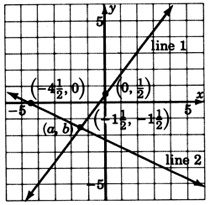The lines are parallel. They do not intersect. The system has no solution.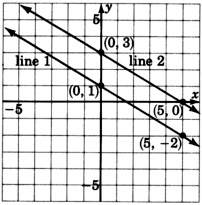The lines are coincident (one on the other). They intersect at infinitely many points. The system has infinitely many solutions.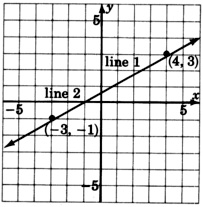## Independent systems

Systems in which the lines intersect at precisely one point are called independent systems . In applications, independent systems can arise when the collected data are accurate and complete. For example,

The sum of two numbers is 10 and the product of the two numbers is 21. Find the numbers.

In this application, the data are accurate and complete. The solution is 7 and 3.

## Inconsistent systems

Systems in which the lines are parallel are called inconsistent systems . In applications, inconsistent systems can arise when the collected data are contradictory. For example,

The sum of two even numbers is 30 and the difference of the same two numbers is 0. Find the numbers.

The data are contradictory. There is no solution to this application.

## Dependent systems

Systems in which the lines are coincident are called dependent systems . In applications, dependent systems can arise when the collected data are incomplete. For example.

The difference of two numbers is 9 and twice one number is 18 more than twice the other.

The data are incomplete. There are infinitely many solutions.

## The method of solving a system graphically

To solve a system of equations graphically: Graph both equations.
1. If the lines intersect, the solution is the ordered pair that corresponds to the point of intersection. The system is independent.
2. If the lines are parallel, there is no solution. The system is inconsistent.
3. If the lines are coincident, there are infinitely many solutions. The system is dependent.

## Sample set a

Solve each of the following systems by graphing.

$\left\{\begin{array}{ccc}\begin{array}{r}2x+y=5\\ x+y=2\end{array}& & \begin{array}{l}\left(1\right)\\ \left(2\right)\end{array}\end{array}$
Write each equation in slope-intercept form.

$\begin{array}{lllllll}\left(1\right)\hfill & \hfill & -2x+y=5\hfill & \hfill & \left(2\right)\hfill & \hfill & x+y=2\hfill \\ \hfill & \hfill & y=2x+5\hfill & \hfill & \hfill & \hfill & y=-x+2\hfill \end{array}$
Graph each of these equations.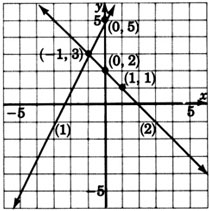The lines appear to intersect at the point $\left(-1,\text{\hspace{0.17em}}3\right)$ . The solution to this system is $\left(-1,\text{\hspace{0.17em}}3\right)$ , or

$\begin{array}{ccc}x=-1,& & y=3\end{array}$

Check:   Substitute $x=-1,\text{\hspace{0.17em}}y=3$ into each equation.

$\begin{array}{llllllllllll}\left(1\right)\hfill & \hfill & \hfill -2x+y& =\hfill & 5\hfill & \hfill & \left(2\right)\hfill & \hfill & \hfill x+y& =\hfill & 2\hfill & \hfill \\ \hfill & \hfill & \hfill -2\left(-1\right)+3& =\hfill & 5\hfill & \text{Is\hspace{0.17em}this\hspace{0.17em}correct?}\hfill & \hfill & \hfill & \hfill -1+3& =\hfill & 2\hfill & \text{Is\hspace{0.17em}this\hspace{0.17em}correct?}\hfill \\ \hfill & \hfill & \hfill 2+3& =\hfill & 5\hfill & \text{Is\hspace{0.17em}this\hspace{0.17em}correct?}\hfill & \hfill & \hfill & \hfill 2& =\hfill & 2\hfill & \text{Yes,\hspace{0.17em}this\hspace{0.17em}is\hspace{0.17em}correct}\text{.}\hfill \\ \hfill & \hfill & \hfill 5& =\hfill & 5\hfill & \text{Yes,\hspace{0.17em}this\hspace{0.17em}is\hspace{0.17em}correct}\text{.}\hfill & \hfill & \hfill & \hfill & \hfill & \hfill & \hfill \end{array}$

$\left\{\begin{array}{ccc}\begin{array}{l}-x+y=-1\\ -x+y=2\end{array}& & \begin{array}{l}\left(1\right)\\ \left(2\right)\end{array}\end{array}$
Write each equation in slope-intercept form.

$\begin{array}{rrrrrrrrrrr}\hfill \left(1\right)& \hfill & \hfill -x+y& \hfill =& -1\hfill & \hfill & \hfill \left(2\right)& \hfill & \hfill -x+y& \hfill =& 2\hfill \\ \hfill & \hfill & \hfill y& \hfill =& x-1\hfill & \hfill & \hfill & \hfill & \hfill y& \hfill =& x+2\hfill \end{array}$
Graph each of these equations.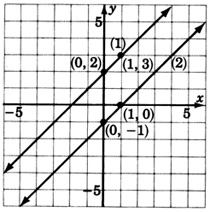These lines are parallel. This system has no solution. We denote this fact by writing inconsistent .

We are sure that these lines are parallel because we notice that they have the same slope, $m=1$ for both lines. The lines are not coincident because the $y$ -intercepts are different.

$\left\{\begin{array}{ccc}\begin{array}{l}-2x+3y=-2\\ -6x+9y=-6\end{array}& & \begin{array}{l}\left(1\right)\\ \left(2\right)\end{array}\end{array}$
Write each equation in slope-intercept form.

$\begin{array}{rrrrrrrrrrr}\hfill \left(1\right)& \hfill & \hfill -2x+3y& \hfill =& -2\hfill & \hfill & \hfill \left(2\right)& \hfill & \hfill -6x+9y& \hfill =& -6\hfill \\ \hfill & \hfill & \hfill 3y& \hfill =& 2x-2\hfill & \hfill & \hfill & \hfill & \hfill 9y& \hfill =& 6x-6\hfill \\ \hfill & \hfill & \hfill y& \hfill =& \hfill \frac{2}{3}x-\frac{2}{3}& \hfill & \hfill & \hfill & \hfill y& \hfill =& \hfill \frac{2}{3}x-\frac{2}{3}\end{array}$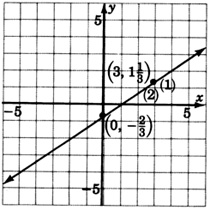Both equations are the same. This system has infinitely many solutions. We write dependent .

## Practice set a

Solve each of the following systems by graphing. Write the ordered pair solution or state that the system is inconsistent, or dependent.

$\left\{\begin{array}{l}2x+y=1\\ -x+y=-5\end{array}$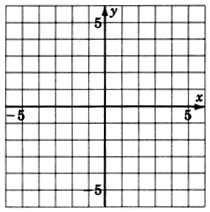$x=2,y=-3$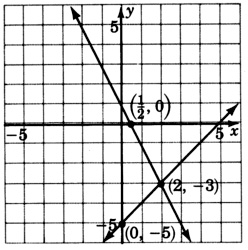$\left\{\begin{array}{l}-2x+3y=6\\ 6x-9y=-18\end{array}$dependent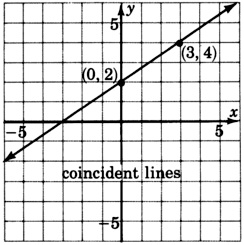$\left\{\begin{array}{l}3x+5y=15\\ 9x+15y=15\end{array}$inconsistent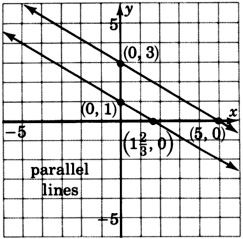$\left\{\begin{array}{r}y=-3\\ x+2y=-4\end{array}$$x=2,y=-3$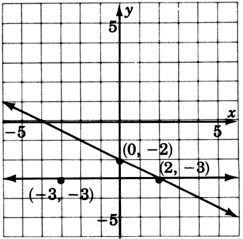## Exercises

For the following problems, solve the systems by graphing. Write the ordered pair solution, or state that the system is inconsistent or dependent.

$\left\{\begin{array}{l}\text{\hspace{0.17em}}\text{\hspace{0.17em}}x+y=-5\\ -x+y=\text{\hspace{0.17em}}\text{\hspace{0.17em}}\text{\hspace{0.17em}}1\end{array}$$\left(-3,-2\right)$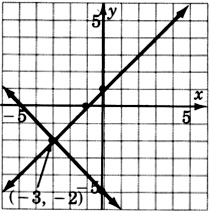$\left\{\begin{array}{l}x+y=4\\ x+y=0\end{array}$$\left\{\begin{array}{l}-3x+y=5\\ -\text{\hspace{0.17em}}\text{\hspace{0.17em}}x+y=3\end{array}$$\left(-1,2\right)$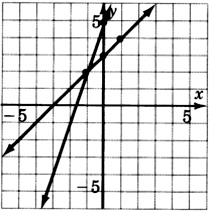$\left\{\begin{array}{l}x-y\text{\hspace{0.17em}}\text{\hspace{0.17em}}\text{\hspace{0.17em}}=-6\\ x\text{\hspace{0.17em}}+2y=\text{\hspace{0.17em}}\text{\hspace{0.17em}}\text{\hspace{0.17em}}0\end{array}$$\left\{\begin{array}{l}3x+\text{\hspace{0.17em}}\text{\hspace{0.17em}}\text{\hspace{0.17em}}y=0\\ 4x-3y=\text{\hspace{0.17em}}12\end{array}$$\left(\frac{12}{13},-\frac{36}{13}\right)$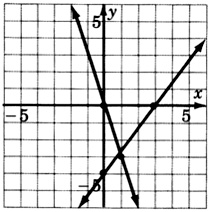$\left\{\begin{array}{l}-4x+\text{\hspace{0.17em}}y=7\\ -3x+\text{\hspace{0.17em}}y=2\end{array}$$\left\{\begin{array}{l}2x+3y=\text{\hspace{0.17em}}6\\ 3x+4y=\text{\hspace{0.17em}}6\end{array}$These coordinates are hard to estimate. This problem illustrates that the graphical method is not always the most accurate. $\left(-6,6\right)$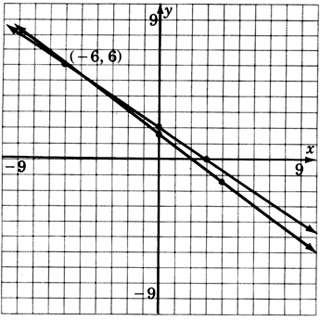$\left\{\begin{array}{l}\text{\hspace{0.17em}}\text{\hspace{0.17em}}x+\text{\hspace{0.17em}}\text{\hspace{0.17em}}\text{\hspace{0.17em}}y=-3\\ 4x+\text{\hspace{0.17em}}4y=-12\end{array}$$\left\{\begin{array}{l}2x-3y=1\\ 4x-6y=4\end{array}$inconsistent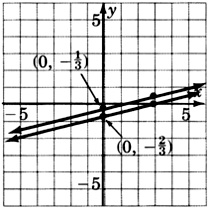$\left\{\begin{array}{l}\text{\hspace{0.17em}}\text{\hspace{0.17em}}\text{\hspace{0.17em}}\text{\hspace{0.17em}}x+2y=\text{\hspace{0.17em}}\text{\hspace{0.17em}}\text{\hspace{0.17em}}3\\ -3x-6y=-9\end{array}$$\left\{\begin{array}{l}\text{\hspace{0.17em}}\text{\hspace{0.17em}}x-2y=\text{\hspace{0.17em}}\text{\hspace{0.17em}}6\\ 3x-6y=18\end{array}$dependent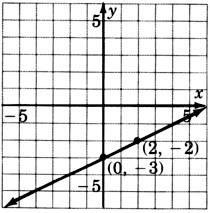$\left\{\begin{array}{l}\text{\hspace{0.17em}}\text{\hspace{0.17em}}\text{\hspace{0.17em}}\text{\hspace{0.17em}}2x\text{\hspace{0.17em}}+3y=\text{\hspace{0.17em}}\text{\hspace{0.17em}}\text{\hspace{0.17em}}\text{\hspace{0.17em}}6\\ -10x-15y=30\end{array}$## Exercises for review

( [link] ) Express $0.000426$ in scientific notation.

$4.26×{10}^{-4}$

( [link] ) Find the product: ${\left(7x-3\right)}^{2}.$

( [link] ) Supply the missing word. The of a line is a measure of the steepness of the line.

slope

( [link] ) Supply the missing word. An equation of the form $a{x}^{2}+bx+c=0,a\ne 0$ , is called a equation.

( [link] ) Construct the graph of the quadratic equation $y={x}^{2}-3.$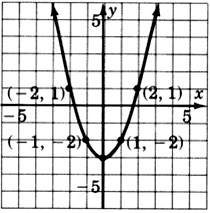how can chip be made from sand
are nano particles real
yeah
Joseph
Hello, if I study Physics teacher in bachelor, can I study Nanotechnology in master?
no can't
Lohitha
where we get a research paper on Nano chemistry....?
nanopartical of organic/inorganic / physical chemistry , pdf / thesis / review
Ali
what are the products of Nano chemistry?
There are lots of products of nano chemistry... Like nano coatings.....carbon fiber.. And lots of others..
learn
Even nanotechnology is pretty much all about chemistry... Its the chemistry on quantum or atomic level
learn
da
no nanotechnology is also a part of physics and maths it requires angle formulas and some pressure regarding concepts
Bhagvanji
hey
Giriraj
Preparation and Applications of Nanomaterial for Drug Delivery
revolt
da
Application of nanotechnology in medicine
has a lot of application modern world
Kamaluddeen
yes
narayan
what is variations in raman spectra for nanomaterials
ya I also want to know the raman spectra
Bhagvanji
I only see partial conversation and what's the question here!
what about nanotechnology for water purification
please someone correct me if I'm wrong but I think one can use nanoparticles, specially silver nanoparticles for water treatment.
Damian
yes that's correct
Professor
I think
Professor
Nasa has use it in the 60's, copper as water purification in the moon travel.
Alexandre
nanocopper obvius
Alexandre
what is the stm
is there industrial application of fullrenes. What is the method to prepare fullrene on large scale.?
Rafiq
industrial application...? mmm I think on the medical side as drug carrier, but you should go deeper on your research, I may be wrong
Damian
How we are making nano material?
what is a peer
What is meant by 'nano scale'?
What is STMs full form?
LITNING
scanning tunneling microscope
Sahil
how nano science is used for hydrophobicity
Santosh
Do u think that Graphene and Fullrene fiber can be used to make Air Plane body structure the lightest and strongest. Rafiq
Rafiq
what is differents between GO and RGO?
Mahi
what is simplest way to understand the applications of nano robots used to detect the cancer affected cell of human body.? How this robot is carried to required site of body cell.? what will be the carrier material and how can be detected that correct delivery of drug is done Rafiq
Rafiq
if virus is killing to make ARTIFICIAL DNA OF GRAPHENE FOR KILLED THE VIRUS .THIS IS OUR ASSUMPTION
Anam
analytical skills graphene is prepared to kill any type viruses .
Anam
Any one who tell me about Preparation and application of Nanomaterial for drug Delivery
Hafiz
what is Nano technology ?
write examples of Nano molecule?
Bob
The nanotechnology is as new science, to scale nanometric
brayan
nanotechnology is the study, desing, synthesis, manipulation and application of materials and functional systems through control of matter at nanoscale
Damian
how did you get the value of 2000N.What calculations are needed to arrive at it
Privacy Information Security Software Version 1.1a
Good
Please keep in mind that it's not allowed to promote any social groups (whatsapp, facebook, etc...), exchange phone numbers, email addresses or ask for personal information on QuizOver's platform.

#### Get Jobilize Job Search Mobile App in your pocket Now!ByByBy Nicole DuquetteBy Briana HamiltonBy Mistry BhaveshBy Sandhills MLTBy RhodesBy Saylor FoundationBy Angela JanuaryBy John GabrieliBy OpenStaxBy Madison Christian